[ITOC-ch1] REGULAR LANGUAGES

1.1 FINITE AUTOMATA

Deterministic Finite Automata

• DEF
• Every state of a DFA always has exactly one exiting transition arrow for each symbol in the alphabet.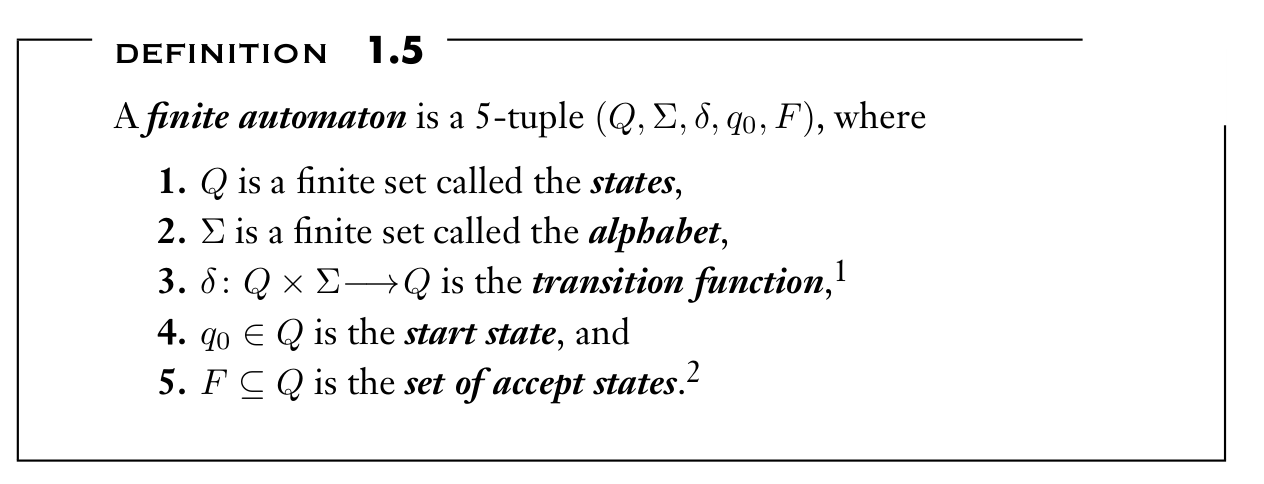Computation under DFA

DEF

• Let M = (Q, Σ, δ, q0 , F ) be a finite automaton and let w = $w_1 w_2 · · · w_n$ be a string where each $w_i$ is a member of the alphabet Σ.
• Then M accepts w if a sequence of states $r_0 , r_1 , . . . , r_n$ in Q exists with three conditions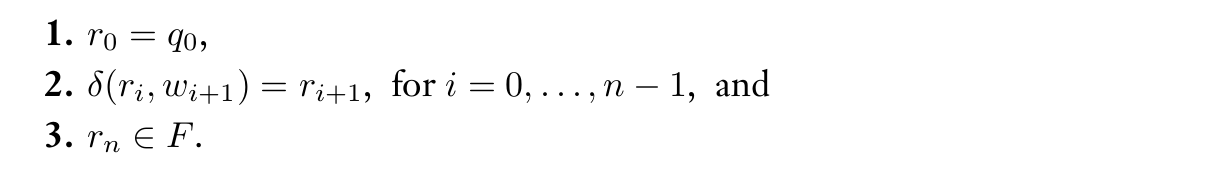• doing computation == state transition

1.2 NONDETERMINISM

Nondeterministic Finite Automata

• DEF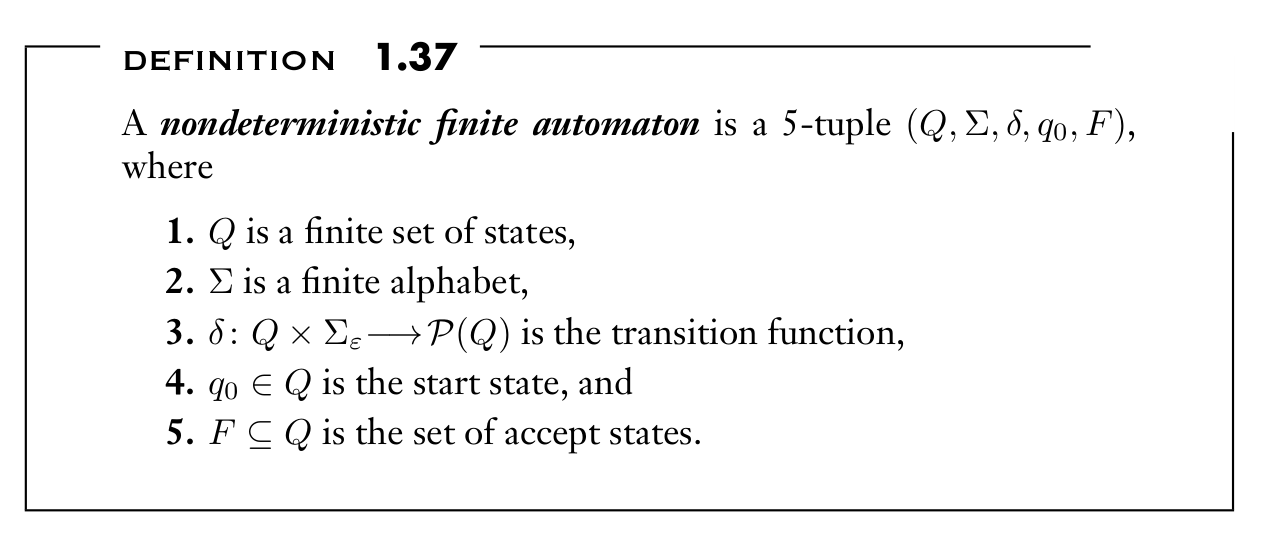Difference

• a state may have ε, zero, one, or many exiting arrows for each alphabet symbol.

Computation under NFA

• ( $\in$ )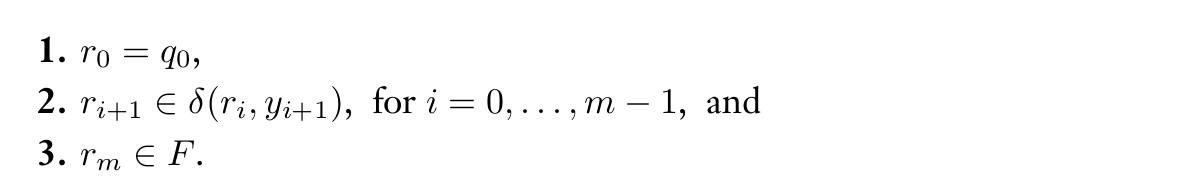EQUIVALENCE OF NFAS AND DFAS

Every nondeterministic finite automaton has an equivalent deterministic finite automaton.

Proof

• convert the NFA into an equivalent DFA that simulates the NFA

We say that M recognizes language A if $A = \{w| M \ accepts \ w \}$

Regular language

DEF

• DFA == NFA == RL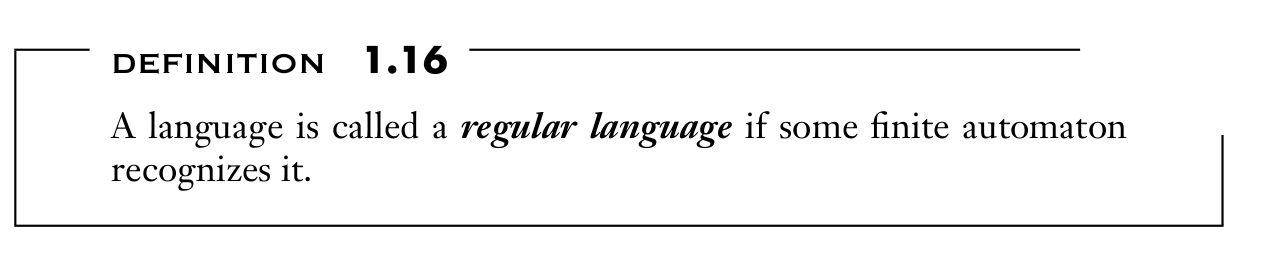Regular Operations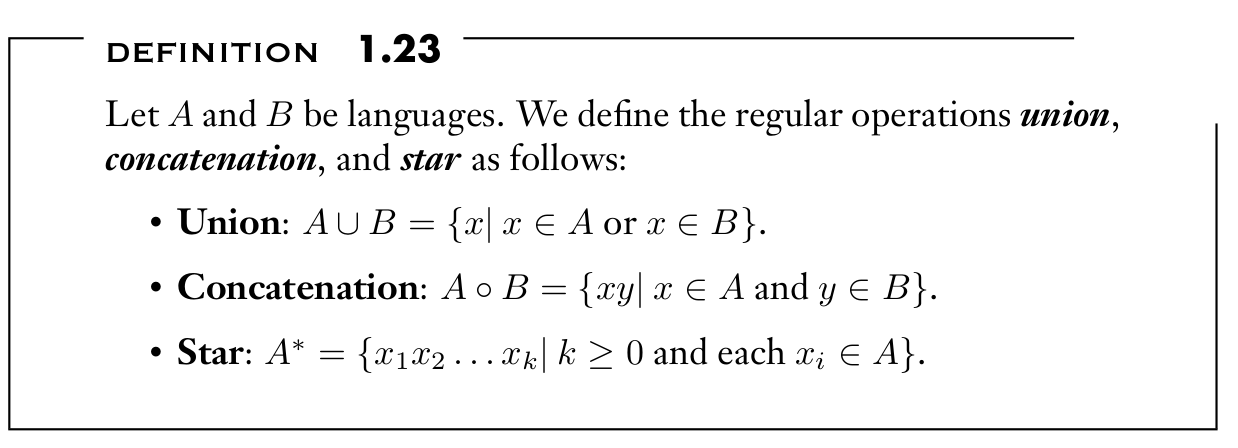CLOSURE UNDER THE REGULAR OPERATIONS

Union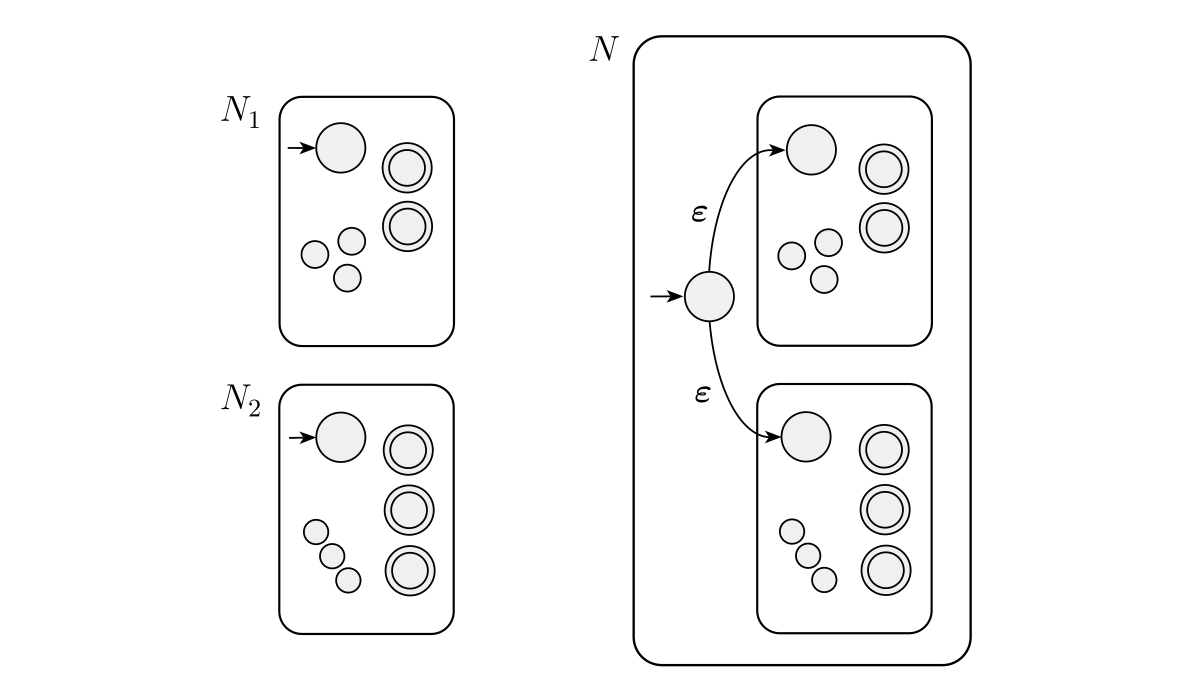Concatenation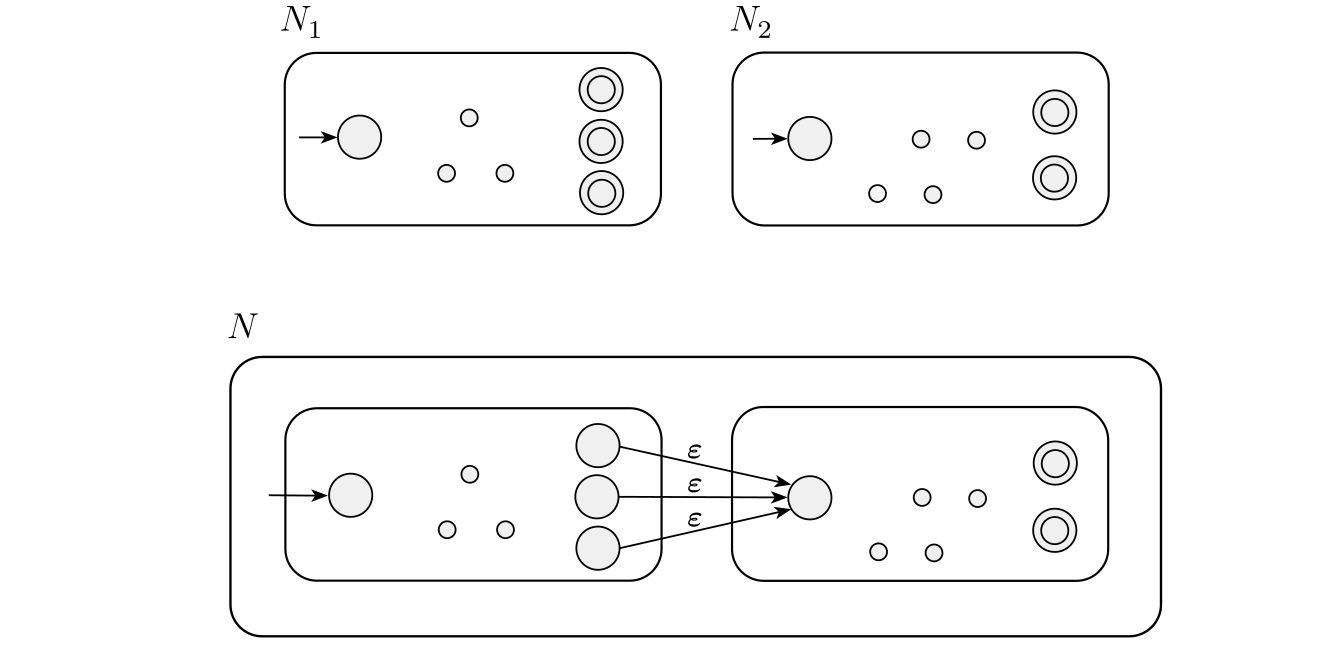Star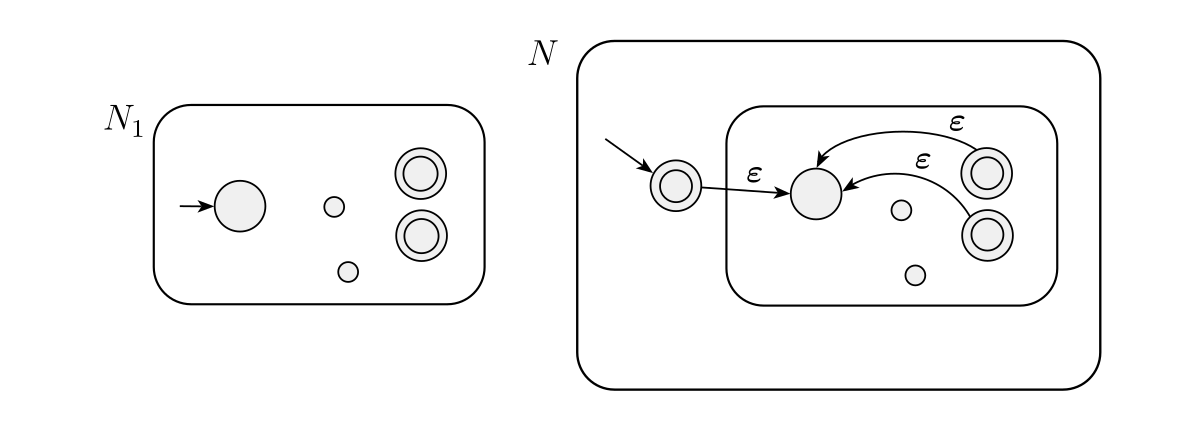1.3 REGULAR EXPRESSIONS

DEF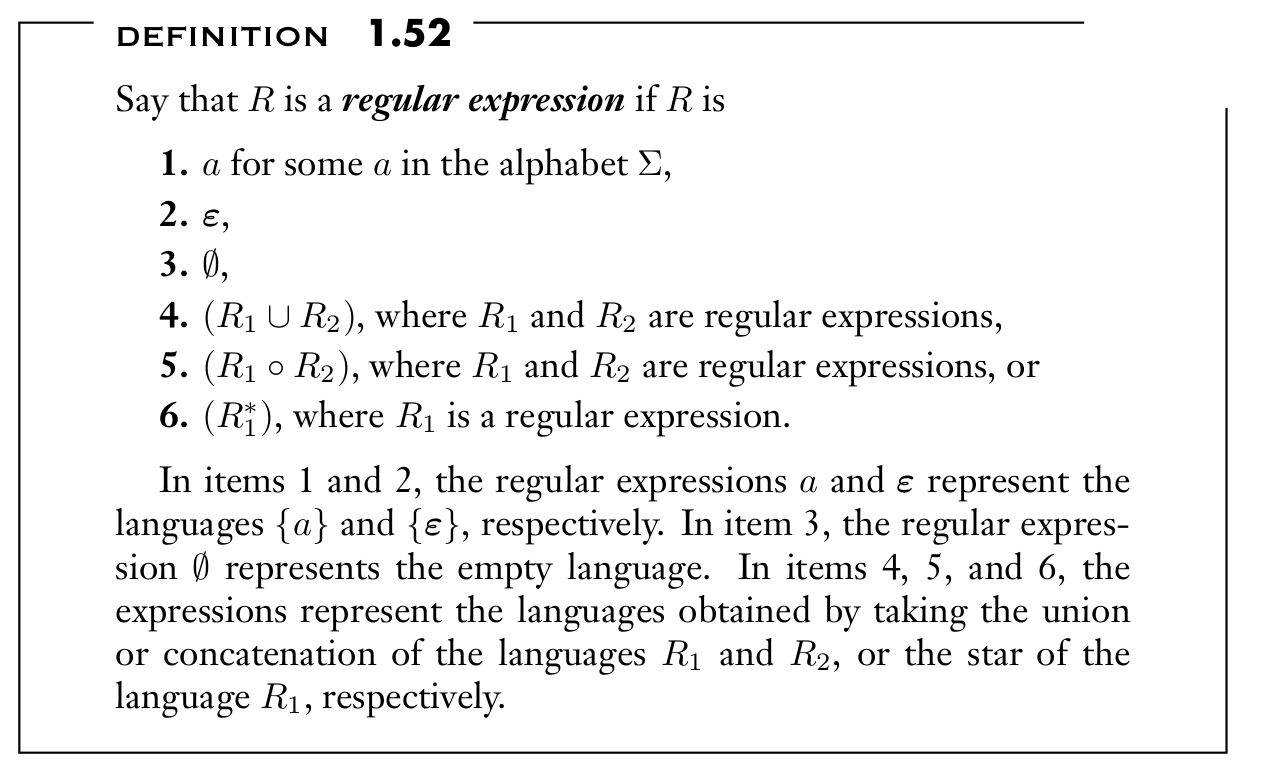$ε$ : empty string;

$∅$ : empty set ( even with out empty string )

$∅ ^ * = {ε}$

$1 ^ ∅ = ∅$

$1 ^ ε = 1 ^ *$

EQUIVALENCE WITH FINITE AUTOMATA

FA == RL == RE

Proof

 If a language is described by a regular expression, then it is regular.

(Proof by recursive definition from base case 1,2,3 )

 If a language is regular, then it is described by a regular expression.

Using GNFA

• the transition arrows may have any regular expressions as labels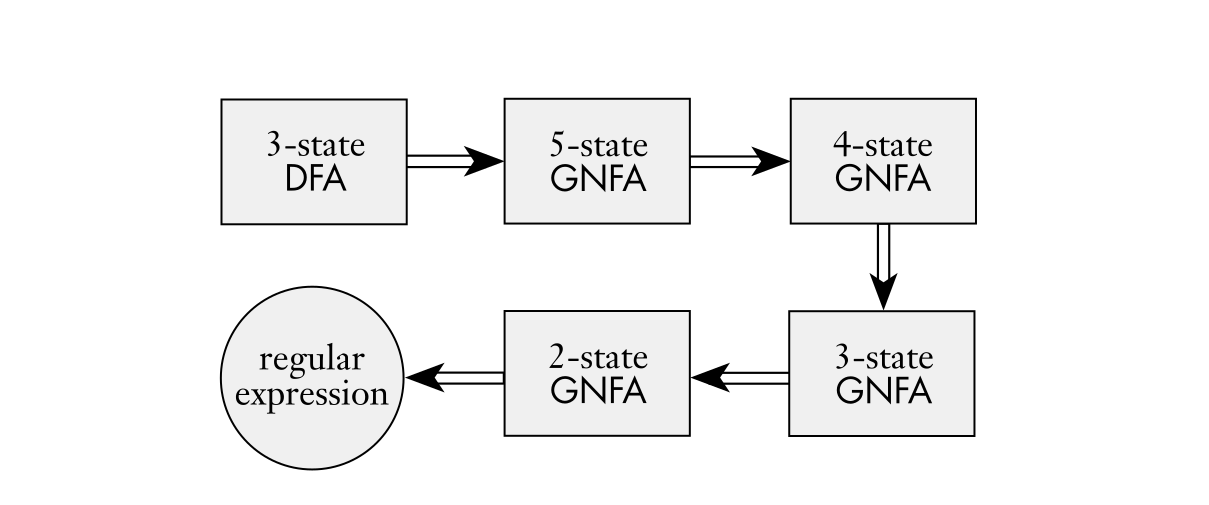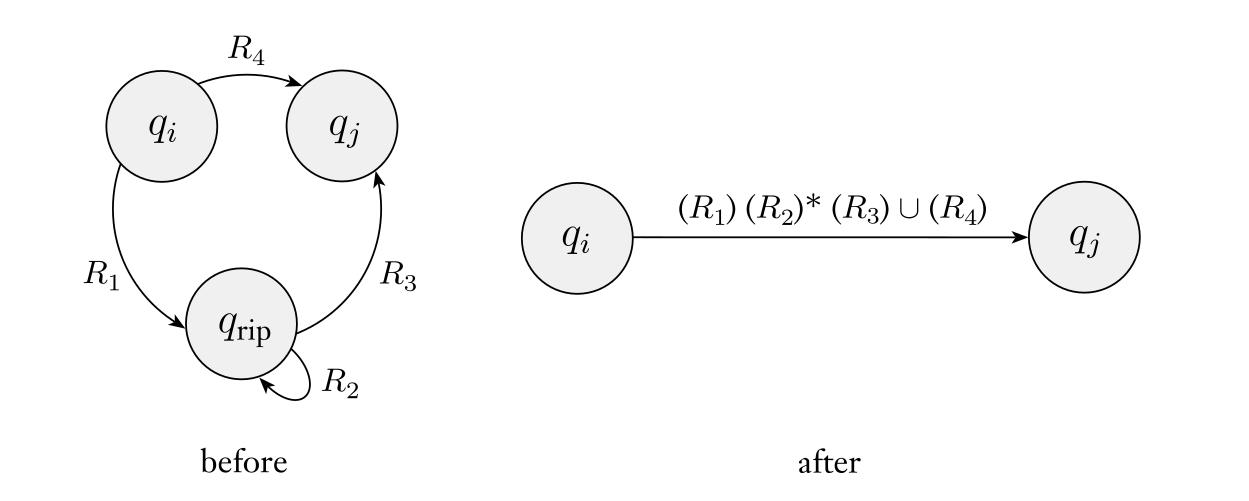1.4 NONREGULAR LANGUAGES

( cannot compute infinite possibilities with finite states )

THE PUMPING LEMMA FOR REGULAR LANGUAGES

DEF

• If A is a regular language, then there is a number p (the pumping length) where if s is any string in A of length at least p, then s may be divided into three pieces, $s = xyz$, satisfying the following conditions: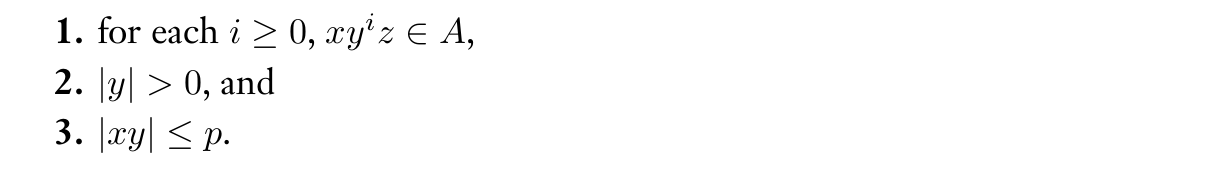Proof

• Let M = DFA that recognizes A. We assign the pumping length p to be the number of states of M.
• We show that any strings in A of length at least p may be broken into the three pieces xyz, satisfying our three conditions.
• Due to pigeon hole principle, among the first p + 1 elements in the sequence, two must be the same state
• Therefore we can divide the string as the following figure while q9 is the state appears twice in the first p+1 length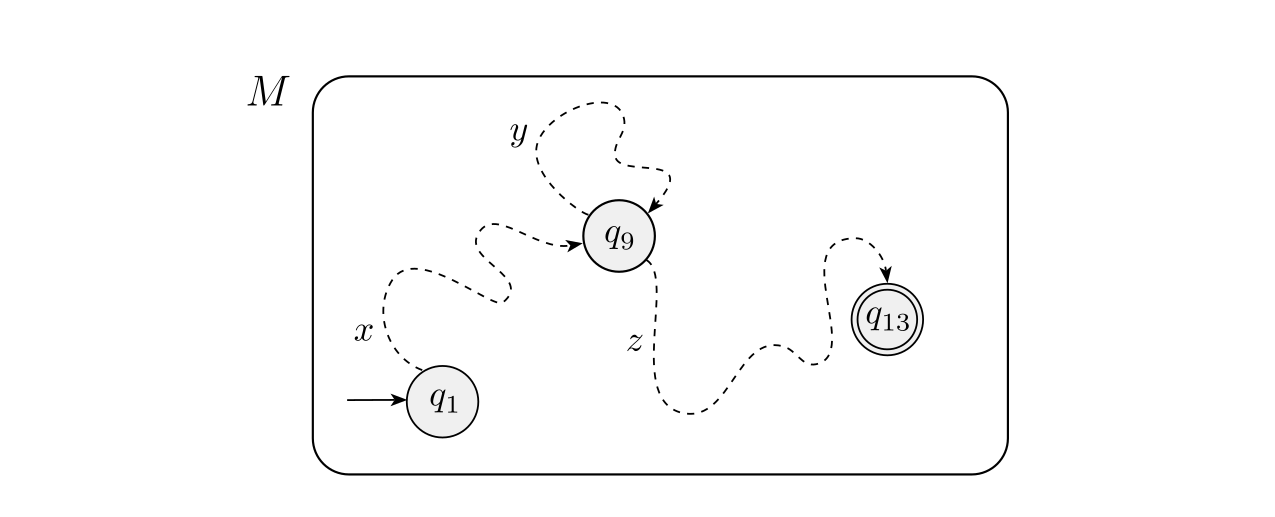Proof language B is not regular

3. considering all ways of dividing s into x, y, and z and for each such division, finding a value i where $xy^i z \notin B$
B $= \{ 0^n 1^n | n ≥ 0 \}$
2. Choose s to be the string $0^p 1^p$.
3. divide s ( 3 cases ) and show $xy^i z \notin B$